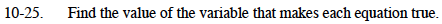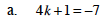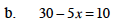### Home > MC1 > Chapter 10 > Lesson 10.1.2 > Problem10-25

10-25.If 4k + 1 = −7, then 4k = −8.
Why does this make sense?

If 4k = −8, what must k be equal to?
Try setting up a diagram to figure it out.

k = −2What do you need to subtract from 30 to get 10?
How can you use this to help figure out the value of x?